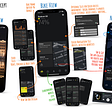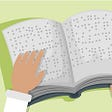# Lightning with Sprite Kit

`void createBolt(float x1, float y1, float x2, float y2, float displace, UIBezierPath *path) {    if (displace < 1.8f) {        CGPoint point = CGPointMake(x2, y2);        [path MoveToPoint:point];    }    else {        float mid_x = (x2+x1)*0.5f;        float mid_y = (y2+y1)*0.5f;        mid_x += (arc4random_uniform(100)*0.01f-0.5f)*displace;        mid_y += (arc4random_uniform(100)*0.01f-0.5f)*displace;        createBolt(x1, y1, mid_x, mid_y, displace*0.5f, path);        createBolt(mid_x, mid_y, x2, y2, displace*0.5f, path);    }}`

# SKShapeNode.

`- (void)addBoltWithStartPoint:(CGPoint)startPoint endPoint:(CGPoint)endPoint {    // Dynamically calculating displace    // Distance between two points    float hypot = hypotf(fabsf(endPoint.x - startPoint.x), fabsf(endPoint.y - startPoint.y));    // hypot/displace = 4/1    float displace = hypot*0.25;     UIBezierPath *path = [UIBezierPath bezierPath];    [path moveToPoint:startPoint];             createBoltPath(startPoint.x, startPoint.y, endPoint.x, endPoint.y, displace, path);     SKShapeNode *bolt = [SKShapeNode node];    bolt.path = path.CGPath;    bolt.strokeColor = [SKColor whiteColor];    bolt.lineWidth = 0.5f;    bolt.antialiased = NO;    [self addChild:bolt];     SKShapeNode *shadowNode = [[SKShapeNode alloc] init];    shadowNode.path = path.CGPath;    shadowNode.strokeColor = [SKColor colorWithRed:0.702 green:0.745 blue:1 alpha:1.0];    shadowNode.lineWidth = 0.5f;    shadowNode.alpha = 0.4;    shadowNode.glowWidth = 5.f;    [self addChild:shadowNode];} - (void)createBoltWithPath:(UIBezierPath*)path {        BoltNode *bolt = [[BoltNode alloc] initWithBezierPath:path lifetime:0.5f];    [self addChild:bolt];}`

# CAShapeLayer.

`- (void)addBoltWithStartPoint:(CGPoint)startPoint endPoint:(CGPoint)endPoint {    // Dynamically calculating displace    // Distance between two points    float hypot = hypotf(fabsf(endPoint.x - startPoint.x), fabsf(endPoint.y - startPoint.y));    // hypot/displace = 4/1    float displace = hypot*0.25;     UIBezierPath *path = [UIBezierPath bezierPath];    [path moveToPoint:startPoint];             createBoltPath(startPoint.x, startPoint.y, endPoint.x, endPoint.y, displace, path);     CAShapeLayer *shapeLayer = [CAShapeLayer layer];    shapeLayer.path = bezierPath.CGPath;    shapeLayer.strokeColor = [[UIColor whiteColor] CGColor];    shapeLayer.lineWidth = 1.f;    shapeLayer.fillColor = [UIColor clearColor].CGColor;    shapeLayer.zPosition = 20;    shapeLayer.shadowColor = [UIColor colorWithRed:0.702 green:0.745 blue:1 alpha:1.0].CGColor;    shapeLayer.shadowOffset = CGSizeMake(0, 0);    shapeLayer.shadowRadius = 7.f;    shapeLayer.shadowOpacity = 1.f;    shapeLayer.shouldRasterize = YES;    [self.view.layer addSublayer:shapeLayer];}`

# SKSpriteNode.

`void createBolt(float x1, float y1, float x2, float y2, float displace, NSMutableArray *pathArray) {    if (displace < 1.8f) {        CGPoint point = CGPointMake(x2, y2);        [pathArray addObject:[NSValue valueWithCGPoint:point]];    }    else {        float mid_x = (x2+x1)*0.5f;        float mid_y = (y2+y1)*0.5f;        mid_x += (arc4random_uniform(100)*0.01f-0.5f)*displace;        mid_y += (arc4random_uniform(100)*0.01f-0.5f)*displace;        createBolt(x1, y1, mid_x, mid_y, displace*0.5f, pathArray);        createBolt(mid_x, mid_y, x2, y2, displace*0.5f, pathArray);    }}`
`@interface LightningLine : SKNode @property (nonatomic) CGPoint startPoint;@property (nonatomic) CGPoint endPoint;@property (nonatomic) float thickness; - (instancetype)initWithStartPoint:(CGPoint)startPoint endPoint:(CGPoint)endPoint;- (void)draw;+ (void)loadSharedAssets; @end @implementation LightningLine - (instancetype)initWithStartPoint:(CGPoint)startPoint endPoint:(CGPoint)endPoint {    if (self = [super init]) {        self.startPoint = startPoint;        self.endPoint = endPoint;        self.thickness = 1.3f;    }    return self;} - (void)draw {    const float imageThickness = 2.f;    float thicknessScale = self.thickness / imageThickness;    CGPoint startPointInThisNode = [self convertPoint:self.startPoint fromNode:self.parent];    CGPoint endPointInThisNode = [self convertPoint:self.endPoint fromNode:self.parent];    float angle = atan2(endPointInThisNode.y - startPointInThisNode.y,                        endPointInThisNode.x - startPointInThisNode.x);    float length = hypotf(fabsf(endPointInThisNode.x - startPointInThisNode.x),                         fabsf(endPointInThisNode.y - startPointInThisNode.y));     SKSpriteNode *halfCircleA = [SKSpriteNode spriteNodeWithTexture:[self halfCircle]];    halfCircleA.anchorPoint = CGPointMake(1, 0.5);    SKSpriteNode *halfCircleB = [SKSpriteNode spriteNodeWithTexture:[self halfCircle]];    halfCircleB.anchorPoint = CGPointMake(1, 0.5);    halfCircleB.xScale = -1.f;    SKSpriteNode *lightningSegment = [SKSpriteNode spriteNodeWithTexture:[self lightningSegment]];    halfCircleA.yScale = halfCircleB.yScale = lightningSegment.yScale = thicknessScale;    halfCircleA.zRotation = halfCircleB.zRotation = lightningSegment.zRotation = angle;    lightningSegment.xScale = length*2;     halfCircleA.blendMode = halfCircleB.blendMode = lightningSegment.blendMode = SKBlendModeAlpha;     halfCircleA.position = startPointInThisNode;    halfCircleB.position = endPointInThisNode;    lightningSegment.position = CGPointMake((startPointInThisNode.x + endPointInThisNode.x)*0.5f,                                            (startPointInThisNode.y + endPointInThisNode.y)*0.5f);    [self addChild:halfCircleA];    [self addChild:halfCircleB];    [self addChild:lightningSegment];} + (void)loadSharedAssets {    static dispatch_once_t onceToken;    dispatch_once(&onceToken, ^{        sHalfCircle = [SKTexture textureWithImageNamed:@"half_circle"];        sLightningSegment = [SKTexture textureWithImageNamed:@"lightning_segment"];    });} static SKTexture *sHalfCircle = nil;- (SKTexture*)halfCircle {    return sHalfCircle;} static SKTexture *sLightningSegment = nil;- (SKTexture*)lightningSegment {    return sLightningSegment;} @end`
`- (instancetype)initWithSize:(CGSize)size {    if (self = [super initWithSize:size]) {        [LightningLine loadSharedAssets];    }    return self;}`
`- (void)drawBoltFromPoint:(CGPoint)startPoint toPoint:(CGPoint)endPoint {    // Dynamically calculating displace    float hypot = hypotf(fabsf(endPoint.x - startPoint.x), fabsf(endPoint.y - startPoint.y));    // hypot/displace = 4/1    float displace = hypot*0.25;    float angle = atan2(endPoint.x - startPoint.x, endPoint.y - startPoint.y);     NSMutableArray *pathArray = [NSMutableArray array];    [pathArray addObject:[NSValue valueWithCGPoint:startPoint]];    createBolt(startPoint.x, startPoint.y, endPoint.x, endPoint.y, displace, pathArray);    //    NSMutableArray *boltLines = [NSMutableArray array];    for (int i = 0; i < pathArray.count - 1; i = i + 1) {        LightningLine *line = [[LightningLine alloc] initWithStartPoint:((NSValue *)pathArray[i]).CGPointValue                                                                endPoint:((NSValue *)pathArray[i+1]).CGPointValue];        [self addChild:line];        [line draw];    }}`

--

--

--

## More from Andrey Gordeev

iOS/Android developer

Love podcasts or audiobooks? Learn on the go with our new app.## Andrey Gordeev

iOS/Android developer

## MVC Design Pattern## VanMoof App UX/UI Concept revisited## Accessibility in iOS [Part I]